Introduction

This package was first designed to set breakpoints for truncating the plot as I need to shrink outlier long branch of a phylogenetic tree.

Axis break or a so call gap plot is useful for large datasets that are not normally distributed and contain outliers. Sometimes we can transform the data (e.g. using log-transformation if the data was log-normal distributed) to solve this problem. But this is not always granted. The data may just simply contain outliers and these outliers are meaningful. A simple gap plot can solve this issue well to present the data in detail with both normal and extreme data.

This package provides several scale functions to break down a ‘gg’ plot into pieces and align them together with (gap plot) or without (wrap plot or cut plot) ignoring subplots. Our methods are fully compatible with ggplot2, so that users can still use the + operator to add geometric layers after creating a broken axis.

If you use ggbreak in published research, please cite the following paper:

• S Xu#, M Chen#, T Feng, L Zhan, L Zhou, G Yu*. Use ggbreak to effectively utilize plotting space to deal with large datasets and outliers. Frontiers in Genetics. 2021, 12:774846. doi: 10.3389/fgene.2021.774846

Gap plot

For creating gap plot, we only provide scale_x_break and scale_y_break functions. Currently, it is not allowed to apply both functions to set breakpoints for both x and y axes. However, multiple breakpoints on a single axis are supported.

Feature 1: Compatible with ggplot2.

After breaking the plot, we can still superpose geometric layers and set themes.

library(ggplot2)
library(ggbreak)
library(patchwork)

set.seed(2019-01-19)
d <- data.frame(x = 1:20,
y = c(rnorm(5) + 4, rnorm(5) + 20, rnorm(5) + 5, rnorm(5) + 22)
)

p1 <- ggplot(d, aes(y, x)) + geom_col(orientation="y")
d2 <- data.frame(x = c(2, 18), y = c(7, 26), label = c("hello", "world"))
p2 <- p1 + scale_x_break(c(7, 17)) +
geom_text(aes(y, x, label=label), data=d2, hjust=1, colour = 'firebrick')  +
xlab(NULL) + ylab(NULL) + theme_minimal()

p1 + p2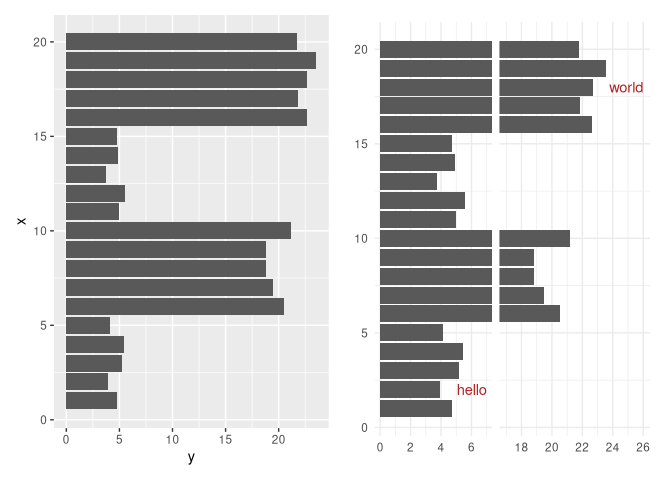Feature 2: Multiple break-points are supported

p2 + scale_x_break(c(18, 21))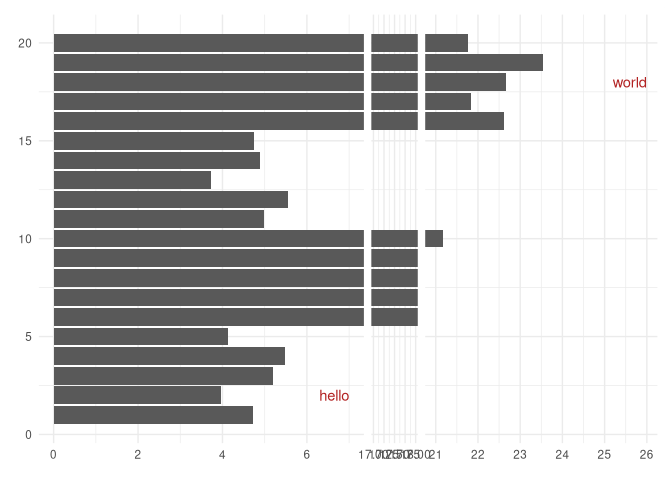Feature 3: Zoom in or zoom out of subplots

p1 + scale_x_break(c(7, 17), scales = 1.5) + scale_x_break(c(18, 21), scales=2)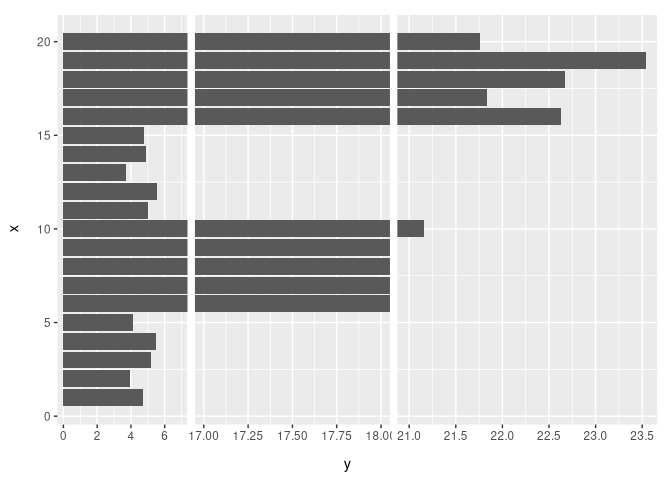Feature 4: Support reverse scale

g <- ggplot(d, aes(x, y)) + geom_col()
g2 <- g + scale_y_break(c(7, 17), scales = 1.5) +
scale_y_break(c(18, 21), scale=2) + scale_y_reverse()
g + g2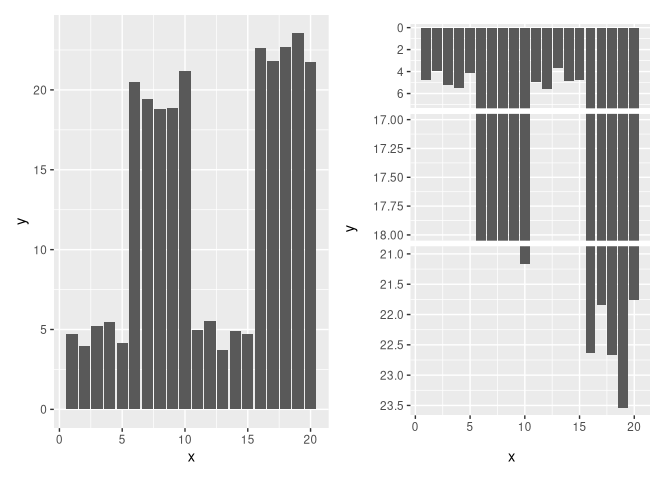Feature 5: Compatible with scale transform functions

Users can apply scale transform functions, such as scale_x_log10 and scale_x_sqrt, to axis break plot.

p2 <- p1 + scale_x_break(c(7, 17))
p3 <- p1 + scale_x_break(c(7, 17)) + scale_x_log10()
p2 + p3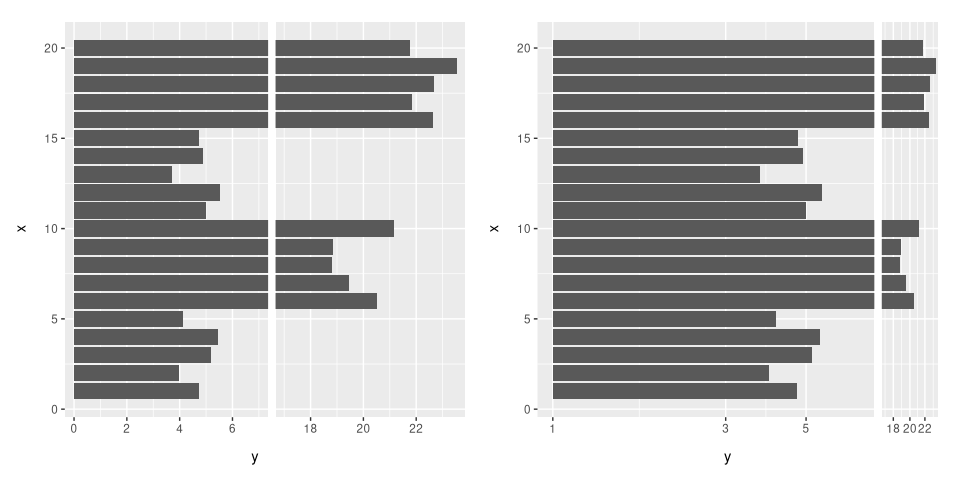Feature 6: Compatible with coord_flip

g + coord_flip() + scale_y_break(c(7, 18))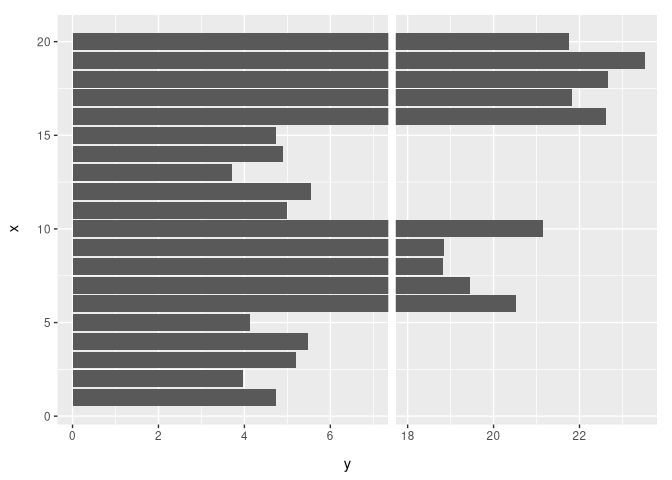Feature 7: Compatible with facet_grid and facet_wrap

set.seed(2019-01-19)
d <- data.frame(
x = 1:20,
y = c(rnorm(5) + 4, rnorm(5) + 20, rnorm(5) + 5, rnorm(5) + 22),
group = c(rep("A", 10), rep("B", 10)),
face=c(rep("C", 5), rep("D", 5), rep("E", 5), rep("F", 5))
)

p <- ggplot(d, aes(x=x, y=y)) +
geom_col(orientation="x") +
scale_y_reverse() +
facet_wrap(group~.,
scales="free_y",
strip.position="right",
nrow=2
) +
coord_flip()
pg <- p +
scale_y_break(c(7, 17), scales="free") +
scale_y_break(c(19, 21), scales="free")
print(pg)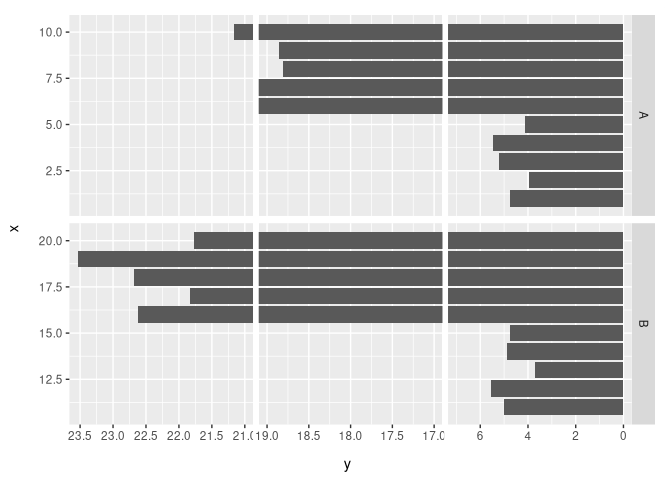Feature 8: Compatible with legends

pg <- pg + aes(fill=group) + theme(legend.position = "bottom")
print(pg)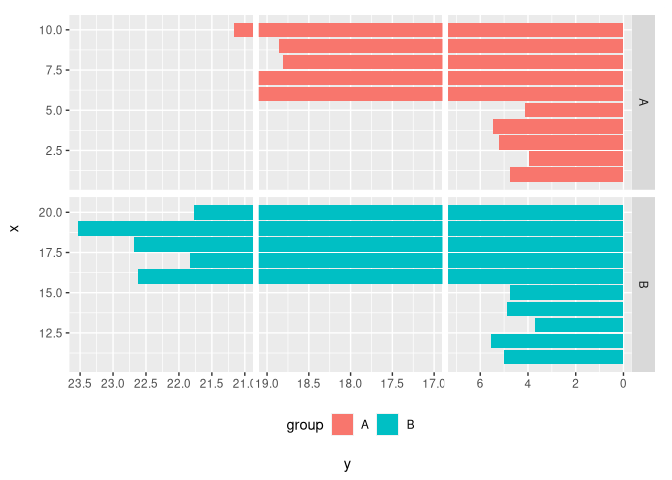Feature 9: Supports all plot labels

pg + labs(title="test title", subtitle="test subtitle", tag="A tag", caption="A caption") +
theme_bw() +
theme(
legend.position = "bottom",
strip.placement = "outside",
axis.title.x=element_text(size=10),
plot.title = element_text(size = 22),
plot.subtitle = element_text(size = 16),
plot.tag = element_text(size = 10),
plot.title.position = "plot",
plot.tag.position = "topright",
plot.caption = element_text(face="bold.italic"),

)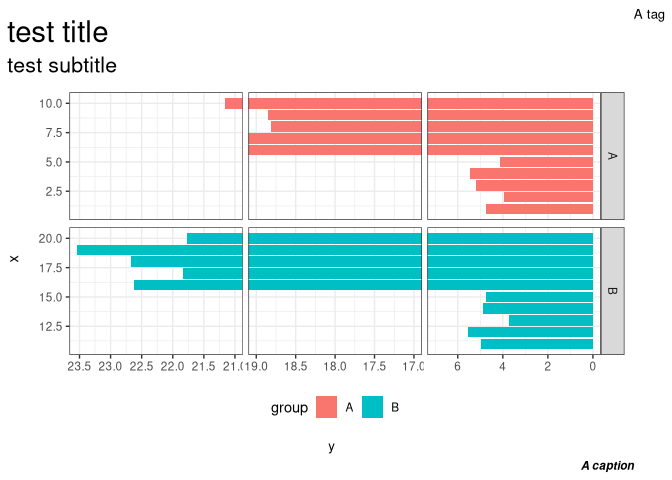Feature 10: Allows setting tick labels for subplots

require(ggplot2)
library(ggbreak)
set.seed(2019-01-19)
d <- data.frame(
x = 1:20,
y =  c(rnorm(5) + 4, rnorm(5) + 20, rnorm(5) + 5, rnorm(5) + 22),
group = c(rep("A", 10), rep("B", 10))
)

p <- ggplot(d, aes(x=x, y=y)) +
scale_y_reverse() +
scale_x_reverse() +
geom_col(aes(fill=group)) +
scale_fill_manual(values=c("#00AED7", "#009E73")) +
facet_wrap(
group~.,
scales="free_y",
strip.position="right",
nrow=2
) +
coord_flip()

p +
scale_y_break(c(7, 10), scales=0.5, ticklabels=c(10, 11.5, 13)) +
scale_y_break(c(13, 17), scales=0.5, ticklabels=c(17, 18, 19)) +
scale_y_break(c(19,21), scales=1, ticklabels=c(21, 22, 23))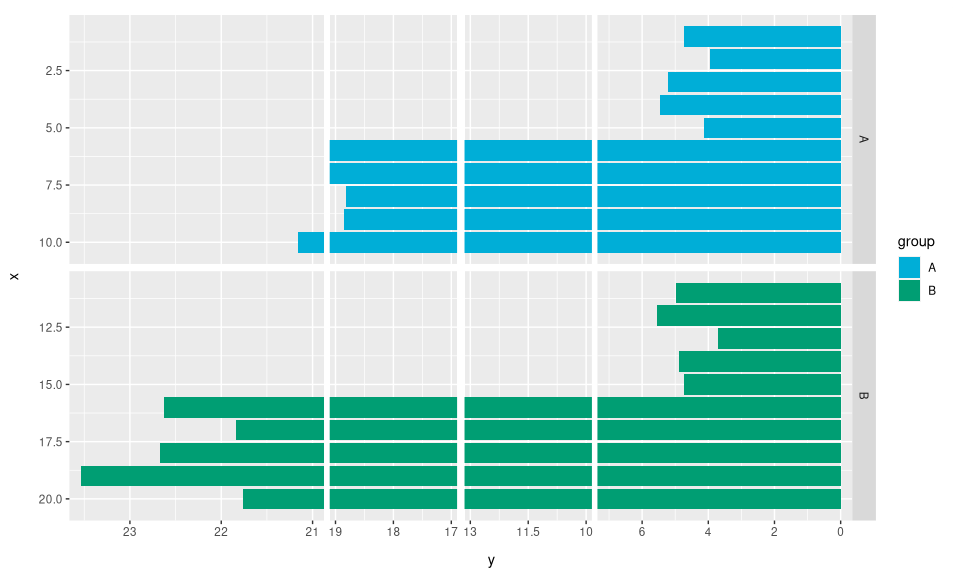Feature 11: Compatible with patchwork

library(patchwork)

set.seed(2019-01-19)
d <- data.frame(
x = 1:20,
y = c(rnorm(5) + 4, rnorm(5) + 20, rnorm(5) + 5, rnorm(5) + 22)
)

p <- ggplot(d, aes(x, y)) + geom_col()
x <- p+scale_y_break(c(7, 17 ))

x + p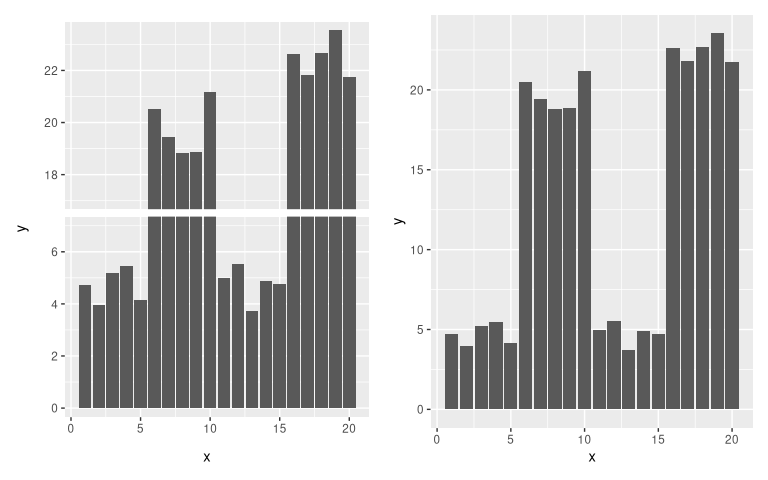Wrap plot

The scale_wrap() function wraps a ‘gg’ plot over multiple rows to make plots with long x-axes easier to read.

p <- ggplot(economics, aes(x=date, y = unemploy, colour = uempmed)) +
geom_line()

p + scale_wrap(n=4)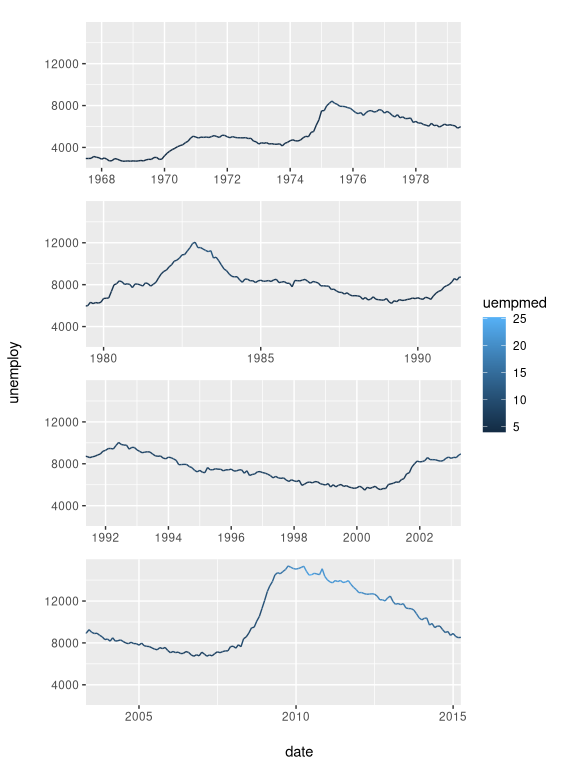Cut plot

The scale_x_cut or scale_y_cut cuts a ‘gg’ plot to several slices with the ability to specify which subplots to zoom in or zoom out.

library(ggplot2)
library(ggbreak)
set.seed(2019-01-19)
d <- data.frame(
x = 1:20,
y = c(rnorm(5) + 4, rnorm(5) + 20, rnorm(5) + 5, rnorm(5) + 22)
)
p <- ggplot(d, aes(x, y)) + geom_col()
p + scale_y_cut(breaks=c(7, 18), which=c(1, 3), scales=c(3, 0.5))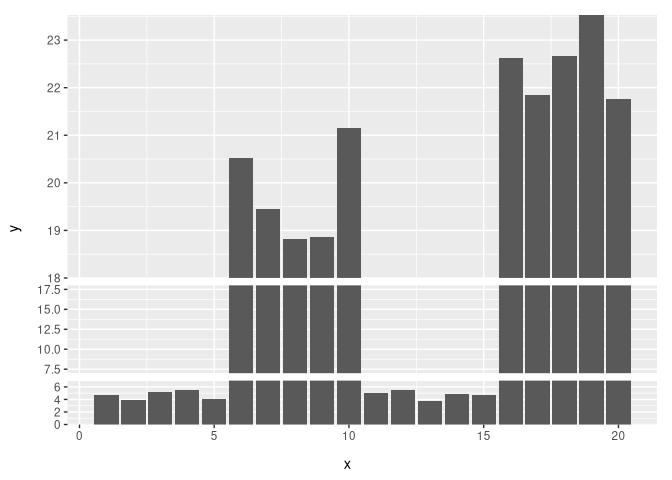Adjust the amount of space between subplots

The space parameter in scale_x_break(), scale_y_break(), scale_x_cut() and scale_y_cut() allows user to control the space between subplots.

p + scale_y_cut(breaks=c(7, 18), which=c(1, 3), scales=c(3, 0.5), space=.5)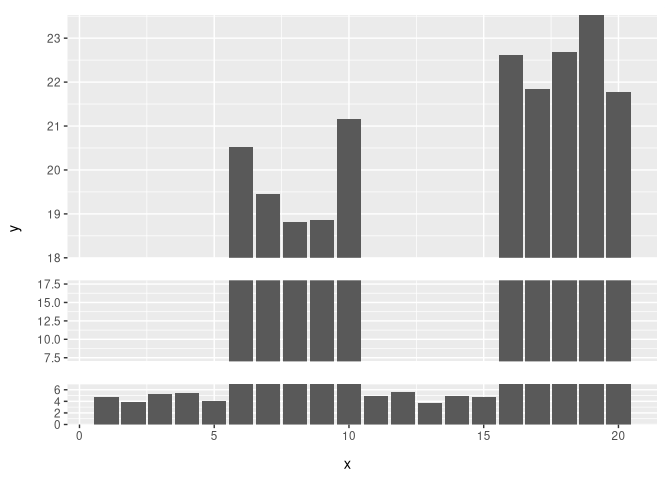Note

The features we introduced for scale_x_break and scale_y_break also work for scale_wrap, scale_x_cut and scale_y_cut.

FAQ

1. Incompatible with functions that arrange multiple plots

You can use aplot::plot_list() to arrange ggbreak objects with other ggplot objects. For other functions, such as cowplot::plot_grid() and gridExtra::grid.arrange(), you need to explictly call print() to ggbreak object, see also https://github.com/YuLab-SMU/ggbreak/issues/36.

Using print() is a secret magic to make ggbreak compatible with other packages, including export.